# Basic Triangle Constructions

Go back to  'Triangles-Quadrilaterals'

We have studied four criteria for congruence of triangles: SAS, ASA, SSS and RHS. For example, in the SAS criterion, if two sides and the included angle of one triangle are correspondingly equal to two sides and the included angle of another, then the two triangles will be congruent. Put another way, we can say that a triangle can be uniquely constructed if two of its sides and the included angle are specified. Similarly, a triangle can be uniquely constructed if:

• one side and two angles are given (ASA or AAS)

• all the three sides are given (SSS)

• the triangle is right-angled, and the hypotenuse and a side are given (RHS)

Let us quickly see how to carry out the construction in each case.

### Case 1 (SAS):

Two sides AB and BC and the included angle, $$\angle B$$, are given.

Draw BC, and through B, a ray inclined to BC at the angle given. On this ray, mark off a point A such that AB is equal to the required length: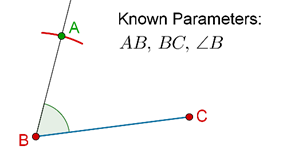Now, join A and C, and the construction will be complete: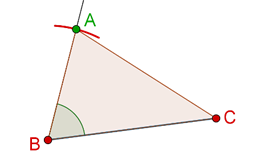### Case 2 (ASA):

Two angles ($$\angle B$$ and $$\angle C$$) and a side (say BC) are given.

Draw BC. Through B and C, draw rays at the specified angles. The point at which these rays intersect is the point A: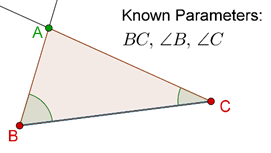$$\Delta ABC$$ is the required triangle. Note that we assumed that the side between the two angles is given. What if we were given $$\angle B$$, $$\angle C$$ and AB (or AC)? How would we have constructed the triangle then?

### Case 3 (SSS):

All the three sides are given.

Draw AB. With A as center, construct an arc with radius equal to AC. With B as center, construct an arc with radius equal to BC. The point of intersection of these two arcs is C: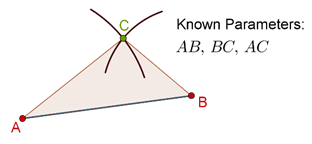$$\Delta ABC$$ is the required triangle.

### Case 4 (RHS):

The triangle is right angled, and the hypotenuse AC and a side (say AB) are given.

Draw AB. Through B, draw a line L perpendicular to AB. Now, with A as center and radius equal to AC, draw an arc to cut L at C: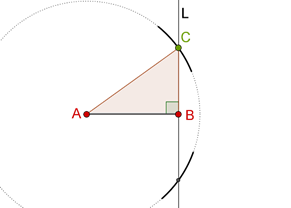$$\Delta ABC$$ is the required triangle. Note that you can cut L in two points this way – but both the triangles so obtained will themselves be congruent, so in a way, you are getting just one triangle.